## Power Frequency Overvoltages in Power Systems:

The Power Frequency Overvoltages occur in large power systems and they are of much concern in EHV systems, i.e. systems of 400 kV and above. The main causes for power frequency and its harmonic overvoltages are

4. #### Saturation in transformers, etc.

Power Frequency Overvoltages harmonics and voltages with frequencies nearer to the operating frequency are caused during tap changing operations, by magnetic or ferro-resonance phenomenon in large power transformers, and by resonating overvoltages due to series capacitors with shunt reactors or transformers.

The duration of these overvoltages may be from one to two cycles to a few seconds depending on the overvoltage protection employed.

### 1.Sudden Load Rejection:

Sudden load rejection on large power systems causes the speeding up of generator intervene to restore normal conditions. But initially both the frequency and voltage increase. The approximate voltage rise, neglecting losses, etc. may be taken as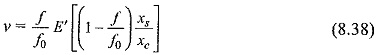where xs is the reactance of the generator (≈ the sum of the transient reactances of the generator and the transformer), xc is the capacitive reactance of the line at open end at increased frequency, E’ the voltage generated before the over-speeding and load rejection, f is the instantaneous increased frequency, and f0 is the normal frequency.

This increase in voltage may go to as high as 2.0 per unit (p.u.) value with 400 kV lines. The voltage at the sending end is affected by the line length, short circuit MVA at sending end bus, and reactive power generation of the line (due to line capacitive reactance and any shunt or series capacitors). Shunt reactors may reduce the voltage to 1.2 to 1.4 p.u.

### 2.Ferranti Effect

Long uncompensated transmission lines exhibit voltage rise at the receiving end. The voltage rise at the receiving end V2 is approximately given by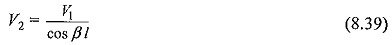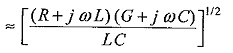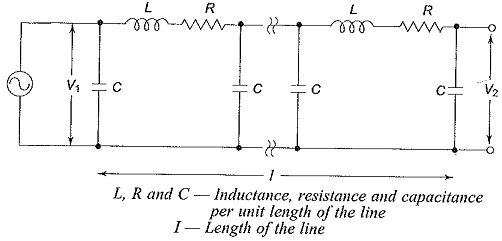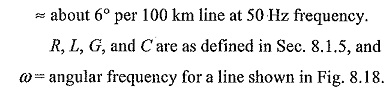Considering that the line capacitance is concentrated at the middle of the line, under open circuit conditions at the receiving end, the line charging current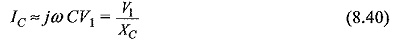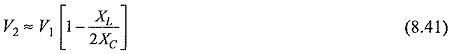where

XL = line inductive reactance, and

XC = line capacitive reactance.

This approximation is shown in Fig. 8.19.

Equation (8.39) gives a better approximation when the line distributed parameters R, L, G, and C per unit length are known.

### 3.Ground Faults and Their Effects

Single line to ground faults cause rise in voltages in other healthy phase. Usually, with solidly grounded systems, the increases in voltage (phase to ground value) will be less than the line-to-line voltage. With effectively ground systems, i.e. with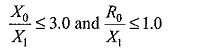(where, R0 and X0 are zero sequence resistance and reactance and X1 is the positive sequence reactance of the system), the rise in voltage of the healthy phases does not usually exceed 1.4 per unit.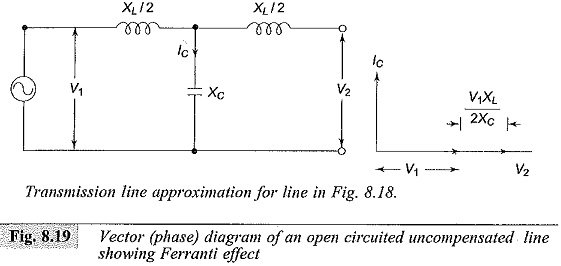### 4.Saturation Effects

When voltages above the rated value are applied to transformers, their magnetizing currents (no load currents also) increase rapidly and may be about the full rated current for 50% overvoltage. These magnetizing currents are not sinusoidal in nature but are of a peaky waveform. The third, fifth, and seventh harmonic contents may be 65%, 35%, and 25% of the exciting current of the fundamental frequency corresponding to an overvoltage of 1.2 p.u. For third and its multiple harmonics, zero sequence impedance values are effective, and delta connected windings suppress them. But the shunt connected capacitors and line capacitances can form resonant circuits and cause high third harmonic overvoltages. When such overvoltages are added, the voltage rise in the lines may be significant. For higher harmonics a series resonance between the transformer inductance and the line capacitance can occur which may produce even higher voltages.